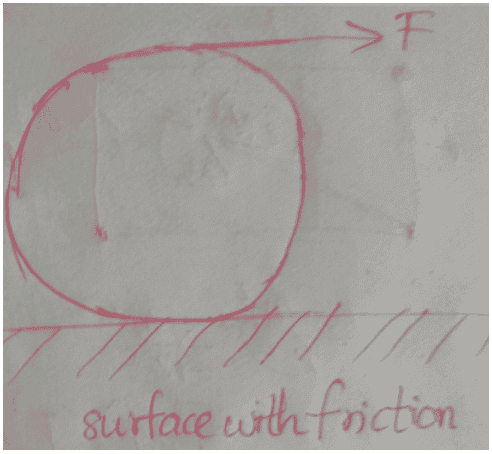# Rotation of a body

## Homework Statement

A force is applied tangentially to a rigid body on a horizontal surface.if it doesnt slip find the frictional force
https://ibb.co/m9sMEU## The Attempt at a Solution

The solution tells us to take axis about the bottommost point in contacy with the surface but why cant i take it through the centre of mass?And can you tell me the direction of friction?

#### Attachments

Last edited by a moderator:

why cant i take it through the centre of mass?

As the force is applied the wheel turns and accelerates to the right. Notice that the axle is not stationary. It accelerates to the right. If you take the axle as your frame of reference, it is an accelerating reference frame. You cannot apply the kinematics you know in a noninertial reference frame.

So say you stay in the reference frame of the ground. At an instant in time the contact point is not moving and the wheel is rotating not about the axle, but rather about the contact point.

haruspex
Homework Helper
Gold Member
If you take the axle as your frame of reference, it is an accelerating reference frame. You cannot apply the kinematics you know in a noninertial reference frame.
Not quite.
In problems such as this, you can choose any axis fixed in an inertial frame OR the mass centre of the object as a moving axis. The reason is that the linear acceleration of the mass has no moment about its mass centre, so whether you use the mass centre as an accelerating axis or the instantaneous position of the mass centre as a fixed axis the equations are the same.

Not quite.
In problems such as this, you can choose any axis fixed in an inertial frame OR the mass centre of the object as a moving axis. The reason is that the linear acceleration of the mass has no moment about its mass centre, so whether you use the mass centre as an accelerating axis or the instantaneous position of the mass centre as a fixed axis the equations are the same.

Yes, I agree that the rotational motion about the axle can be properly expressed in the accelerated reference frame. However it isn’t clear to me how one would infer the friction force in that construction without appealing to linear inertia or in some other way deriving the apparent acceleration of the ground towards the left. I really don’t think it can be done with rotational mechanics alone.

haruspex
Homework Helper
Gold Member
I really don’t think it can be done with rotational mechanics alone.
I did not think that was the point at issue. The OP was only concerned with what was a valid/useful choice of axis for the moments equation.
But now that you mention it, you could take moments about the mass centre to get an equation relating α, F and the frictional force, and moments about the point of contact (as instantaneous centre of rotation) to get an equation relating α to F. Eliminate α and you are home,

I did not think that was the point at issue. The OP was only concerned with what was a valid/useful choice of axis for the moments equation.
But now that you mention it, you could take moments about the mass centre to get an equation relating α, F and the frictional force, and moments about the point of contact (as instantaneous centre of rotation) to get an equation relating α to F. Eliminate α and you are home,
Ok but friction opposes rotation or linear motion?

haruspex
Homework Helper
Gold Member
Ok but friction opposes rotation or linear motion?
Friction opposes relative motion of the surfaces in contact.
For a car going uphill at constant speed, the friction acts up the incline. This promotes linear motion while inhibiting rotational motion; going downhill, the friction still acts up the incline, but now inhibits linear motion and promotes rotational motion.

Not quite.
In problems such as this, you can choose any axis fixed in an inertial frame OR the mass centre of the object as a moving axis. The reason is that the linear acceleration of the mass has no moment about its mass centre, so whether you use the mass centre as an accelerating axis or the instantaneous position of the mass centre as a fixed axis the equations are the same.

The question was “ The solution tells us to take axis about the bottommost point in contacy with the surface but why cant i take it through the centre of mass?“. And your answer is “you can take the center of mass as the axis ... so long as you ALSO take the contact point as the axis” ?!?

haruspex
Homework Helper
Gold Member
The question was “ The solution tells us to take axis about the bottommost point in contacy with the surface but why cant i take it through the centre of mass?“. And your answer is “you can take the center of mass as the axis ... so long as you ALSO take the contact point as the axis” ?!?
No, that is not what I wrote.
In post #3 I wrote that you can take the mass centre as axis instead of using the point of contact. This would be combined with the linear equations in the usual way. I think this answered the OP question.
In post #4, you seemed to think I was saying it was not necessary to use linear analysis at all, and opined that was not possible.
In post #6, I denied I had said that, but pointed out that in fact you could avoid using any linear analysis by using rotational analysis about the two legitimate types of axis: a fixed point and the moving mass centre.

At no point did I claim that a single equation about an astutely chosen axis would suffice.